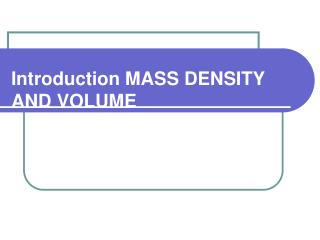DownloadDownload PresentationIntroduction MASS DENSITY AND VOLUME

# Introduction MASS DENSITY AND VOLUME

Télécharger la présentation## Introduction MASS DENSITY AND VOLUME

- - - - - - - - - - - - - - - - - - - - - - - - - - - E N D - - - - - - - - - - - - - - - - - - - - - - - - - - -
##### Presentation Transcript

1. Introduction MASS DENSITY AND VOLUME

2. Measuring Mass • Mass - the quantity of matter in an object • standard unit is gram(g) • Measured using a digital scale or triple beam balance

3. Measuring Volume and Capacity • Volume - the amount of space occupied by an object • standard unit is liter(L) • 1 L = 1000 ml = 1000 cm3 = 1 dm3 • Measured using a graduated cylinder • Capacity - a measure of the volume inside a container

4. Measuring Volume • Measured with a graduated cylinder • Determine value of each mark on the scale • Read scale using the lowest position of the meniscus • Measure the meniscus at eye level from the center of the meniscus. • In the case of water and most liquids, the meniscus is concave. Mercury produces a convex meniscus.

5. Displacement • Displacement • Amount of water an object replaces • Equal to its volume

6. Volume of a Solid, Irregular Object • Displacement - amount of water an object replaces • Procedure • Place graduate beaker beneath spout • Fill the overflow canwith water until water begins to spill • Empty the excess water • Place object to be measured into the overflow can • Remove when water stops flowing out of the can • Measure the displaced water using a graduated cylinder.

7. Volume of a Solid, Irregular Object • Displacement • Calculate the difference between the initial and final volume measurement.

8. Volume of a Solid, Regular Object • Volume -length x width x height • V = 2.8 cm x 3.2 cm x 2.5 cm • V = 22.4 cm3 • Measured with a ruler

9. Calculating Density • Density - a specific property of matter that is related to its mass divided by the volume. • D=M/V • the ratio of mass to volume • used to characterize a substance • each substance has a unique density • Units for density include: • g/mL • g/cm3 • g/cc

10. Measuring Time • Time • metric unit is second (s)

11. Measuring Temperature • Temperature - the degree of “hotness” of an object • standard unit is celsius (°C) • measured with a thermometer

12. Temperature Conversions • Conversion Between Fahrenheit, Celsius, and Kelvin • Example: • Convert 75 ºC to ºF • Convert -10 ºF to ºC

13. Measurement Unit Conversion • You can convert between units of measurement • within the metric system • between the English system and metric system

14. Conversion and the Metric System ACS Ms. Grogan

15. Measurement Unit Conversion • You can convert between units of measurement • within the metric system • between the English system and metric system

16. Unit Conversion • Let your units do the work for you by simply memorizing connections between units. • Example: How many donuts are in one dozen? • We say: “Twelve donuts in a dozen.” • Or: 12 donuts = 1 dozen donuts • What does any number divided by itself equal? • ONE!

17. Unit Conversion • This fraction is called a unit factor • Multiplication by a unit factor does not change the amount - only the unit. • Example:How many donuts are in 3.5 dozen? • You can probably do this in your head but try it using the Factor-Label Method.

18. Unit Conversion Rules • Start with the given information… • Then set up your unit factor… • See that the original unit cancels out… • Then multiply and divide all numbers…

19. Unit Conversion Practice • Example:Convert 12 gallons to units of quarts.

20. Unit Conversion Practice • Example:Convert 4 ounces to kilograms.